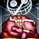# LSMA - A Fast And Simple Alternative Calculation

2472 просмотров
Introduction

At the start of 2019 i published my first post "Approximating A Least Square Moving Average In Pine", who aimed to provide alternatives calculation of the least squares moving average ( LSMA ), a moving average who aim to estimate the underlying trend in the price without excessive lag.

The LSMA has the form of a linear regression ax + b where x is a linear sequence 1.2.3..N and with time varying a and b, the exact formula of the LSMA is as follows :

a = stdev(close,length)/stdev(bar_index,length) * correlation(close,bar_index,length)
b = sma (close,length) - a*sma(bar_index,length)
lsma = a*bar_index + b

Such calculation allow to forecast future values however such forecast is rarely accurate and the LSMA is mostly used as a smoother. In this post an alternative calculation is proposed, such calculation is incredibly simple and allow for an extremely efficient computation of the LSMA .

Rationale

The LSMA is a FIR low-pass filter with the following impulse response :

The impulse response of a FIR filter gives us the weight of the filter, as we can see the weights of the LSMA are a linearly decreasing sequence of values, however unlike the linearly weighted moving average ( WMA ) the weights of the LSMA take on negative values, this is necessary in order to provide a better fit to the data. Based on such impulse response we know that the WMA can help calculate the LSMA , since both have weights representing a linearly decreasing sequence of values, however the WMA doesn't have negative weights, so the process here is to fit the WMA impulse response to the impulse response of the LSMA .

Based on such negative values we know that we must subtract the impulse response of the WMA by a constant value and multiply the result, such constant value can be given by the impulse response of a simple moving average , we must now make sure that the impulse response of the WMA and SMA cross at a precise point, the point where the impulse response of the LSMA is equal to 0.

We can see that 3WMA and 2SMA are equal at a certain point, and that the impulse response of the LSMA is equal to 0 at that point, if we proceed to subtraction we obtain :

Therefore :

LSMA = 3WMA - 2SMA = WMA + 2( WMA - SMA )

Comparison

On a graph the difference isn't visible, subtracting the proposed calculation with a regular LSMA of the same period gives :

the error is 0.0000000...and certainly go on even further, therefore we can assume that the error is due to rounding errors.

Conclusion

This post provided a different calculation of the LSMA , it is shown that the LSMA can be made from the linear combination of a WMA and a SMA : 3WMA + -2SMA. I encourage peoples to use impulse responses in order to estimate other moving averages, since some are extremely heavy to compute.

« Je suis las des cruautés de mes semblables, qui ne sont pas mes pareils.

« Je prendrai l’essor et je m’envolerai vers la mer.

« Je connaîtrai le goût des brises du large. J’entendrai les grands cris de la tempête.

## КомментарииGlad to meet your account. U have post (and resolve) lot's of ideas I have been surfin' on too! Your explanation is much richer than mine, but quite fun to find we end explaining quite the same concept:

Cheers!
ОтветитьHi Alex,
Wow, very incredible indicator! I am no IT nor maths skill, but I love trading with MAs as I find them simple and straight forward.
Although traditional MAs are mainly lagging however, it also depends on how you deploy them on multiple timeframes to make it less lagging.
However, your indicator, in my opinion, has shifted the MAs being lagging to leading indicators as it has smoothed out and hugs very closely to the price action to detect reversal faster . Thanks so much. Its a great help (Y)
ОтветитьYou are the reason that all my codes load fast now, you make stuff just far more efficient. Love it!!
Ответить